## 11.1 – Toolkit Functions

### Learning Objectives

(11.1.1) – Identifying Basic Toolkit Functions

# (11.1.1) – Identifying Basic Toolkit Functions

We will see these toolkit functions, combinations of toolkit functions, their graphs, and their transformations frequently throughout this book. It will be very helpful if we can recognize these toolkit functions and their features quickly by name, formula, graph, and basic table properties. The graphs and sample table values are included with each function shown below.

Toolkit Functions
Name Function Graph
Constant For the constant function $f\left(x\right)=c$,the domain consists of all real numbers; there are no restrictions on the input. The only output value is the constant $c$, so the range is the set $\left\{c\right\}$ that contains this single element. In interval notation, this is written as $\left[c,c\right]$, the interval that both begins and ends with $c$.

Domain: $(-\infty,\infty)$

Range: $[c,c]$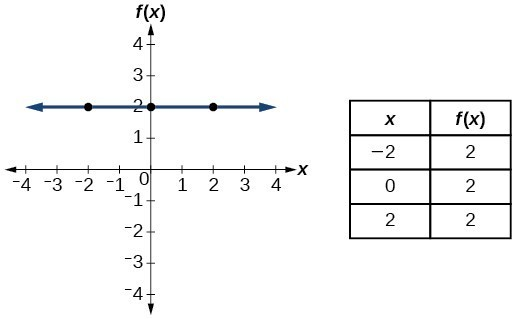Identity For the identity function $f\left(x\right)=x$, there is no restriction on $x$. Both the domain and range are the set of all real numbers.

Domain: $(-\infty,\infty)$

Range: $(-\infty,\infty)$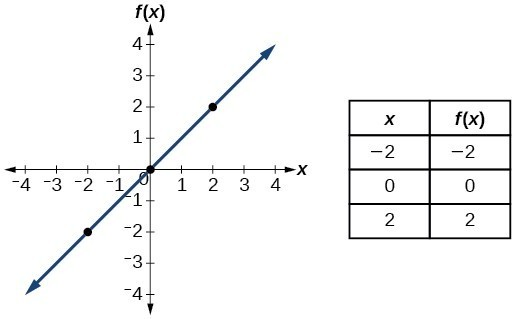Absolute value For the absolute value function $f\left(x\right)=|x|$, there is no restriction on $x$. However, because absolute value is defined as a distance from 0, the output can only be greater than or equal to 0.

Domain: $(-\infty,\infty)$

Range: $[0,\infty)$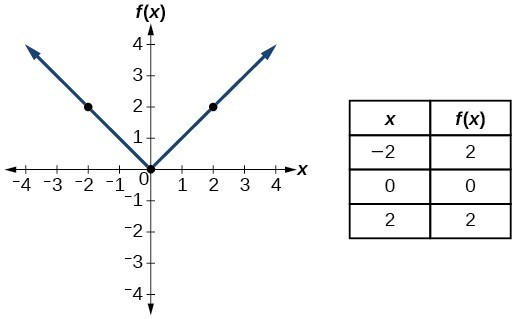Quadratic For the quadratic function $f\left(x\right)={x}^{2}$, the domain is all real numbers since the horizontal extent of the graph is the whole real number line. Because the graph does not include any negative values for the range, the range is only nonnegative real numbers.

Domain: $(-\infty,\infty)$

Range: $[0,\infty)$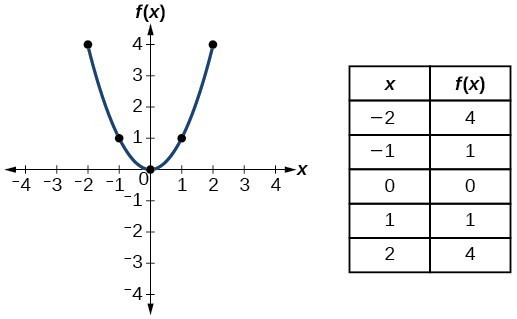Cubic For the cubic function $f\left(x\right)={x}^{3}$, the domain is all real numbers because the horizontal extent of the graph is the whole real number line. The same applies to the vertical extent of the graph, so the domain and range include all real numbers.

Domain: $(-\infty,\infty)$

Range: $(-\infty,\infty)$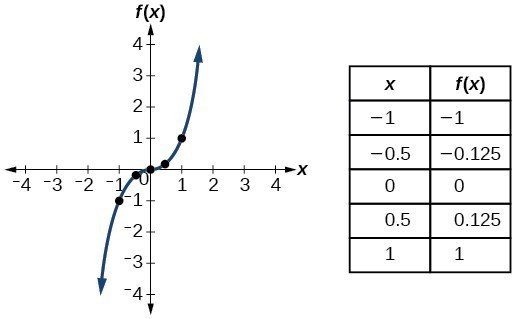Reciprocal/ Rational For the reciprocal function $f\left(x\right)=\frac{1}{x}$, we cannot divide by 0, so we must exclude 0 from the domain. Further, 1 divided by any value can never be 0, so the range also will not include 0. In set-builder notation, we could also write $\left\{x|\text{ }x\ne 0\right\}$, the set of all real numbers that are not zero.

Domain: $(-\infty,0) \cup (0,\infty)$

Range: $(-\infty,0) \cup (0,\infty)$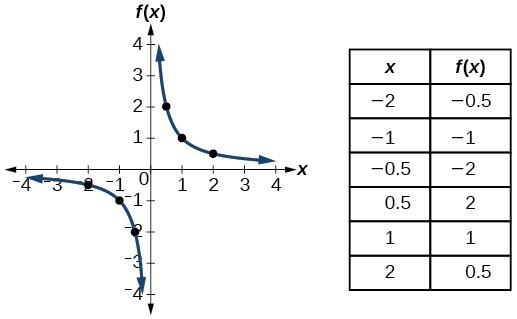Reciprocal / Rational squared For the reciprocal squared function $f\left(x\right)=\frac{1}{{x}^{2}}$, we cannot divide by $0$, so we must exclude $0$ from the domain. There is also no $x$ that can give an output of 0, so 0 is excluded from the range as well. Note that the output of this function is always positive due to the square in the denominator, so the range includes only positive numbers.

Domain: $(-\infty,0) \cup (0,\infty)$

Range: $(0,\infty)$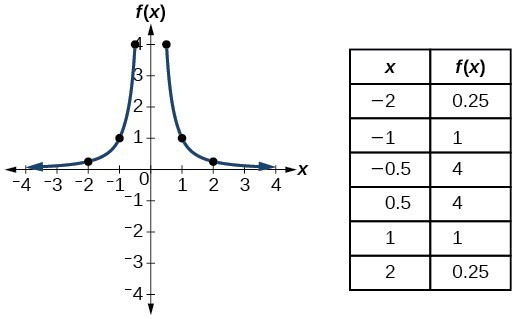Square root  For the square root function $f\left(x\right)=\sqrt[]{x}$, we cannot take the square root of a negative real number, so the domain must be 0 or greater. The range also excludes negative numbers because the square root of a positive number $x$ is defined to be positive, even though the square of the negative number $-\sqrt{x}$ also gives us $x$.

Domain: $[0,\infty)$

Range: $[0,\infty)$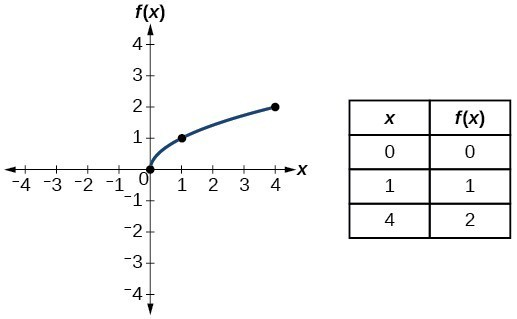Cube root For the cube root function $f\left(x\right)=\sqrt{x}$, the domain and range include all real numbers. Note that there is no problem taking a cube root, or any odd-integer root, of a negative number, and the resulting output is negative (it is an odd function).

Domain: $(-\infty,\infty)$

Range: $(-\infty,\infty)$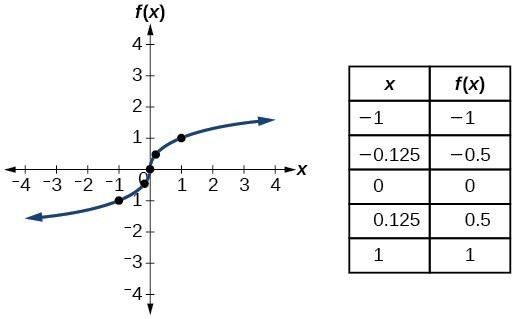### Try It now

In this exercise, you will graph the toolkit functions using Desmos.

1. Graph each toolkit function using function notation.
2. Make a table of values that references the function and includes at least the interval [-5,5].